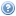# Course List for 2021The undergraduate courses on this list are also available in a timetable view.

Course Title Trimester(s) Teaching Home
COMP 312 Simulation and Stochastic Models 1
DATA 201 Techniques of Data Science 2
DATA 303 Statistics for Data Science 1
DATA 351 Data Science Internship 2
DATA 473 Statistical Modelling for Data Science 1
DATA 480 Research Preparation for Data Science 3
DATA 487 Research Project 1, 3
DATA 489 Research Project 1, 2, 3
DATA 491 Mathematics for Data Science 1
DATA 492 Data Science Algorithms 2
DATA 581 Data Science Practicum 1, 2, 3
DATA 588 Research Project 3
ENGR 123 Engineering Mathematics with Logic and Statistics 2, 3 Home Page
MATH 132 Introduction to Mathematical Thinking 1, 3
MATH 142 Calculus 1B 2
MATH 161 Discrete Mathematics and Logic 2
MATH 243 Multivariable Calculus 2
MATH 245 Computational Mathematics 2
MATH 251 Linear Algebra 2
MATH 301 Differential Equations 2
MATH 309 Mathematical Logic 2
MATH 317 Metric Spaces 1
MATH 318 Hilbert Spaces 2
MATH 335 Computability and Complexity 1
MATH 353 Optimisation 1
MATH 377 Probability and Random Processes 1
MATH 432 Matroid Theory 2
MATH 434 Set Theory 1
MATH 436 Galois Theory and Number Theory 2
MATH 439 Category Theory 1
MATH 441 Measure Theory 1
MATH 443 Operator Algebra 1
MATH 452 Topology 1
MATH 453 Lie Groups and Lie Algebras 1
MATH 461 Differential Equations 1
MATH 465 General Relativity and Cosmology 2
MATH 466 Topics in Applied Mathematics 1
MATH 482 Special Topic: Infinite Combinatorics 1
STAT 193 Statistics in Practice 1, 2, 3
STAT 293 Applied Statistics 2B 2
STAT 332 Statistical Inference 2
STAT 335 Statistical Models for Actuarial Science 1
STAT 391 Mathematical Methods for Applied Statistics 1
STAT 393 Linear Models 2
STAT 394 Multivariate Statistics 2
STAT 431 Biostatistics 1
STAT 432 Computational Statistics 2
STAT 433 Stochastic Processes 2
STAT 434 Statistical Inference 2